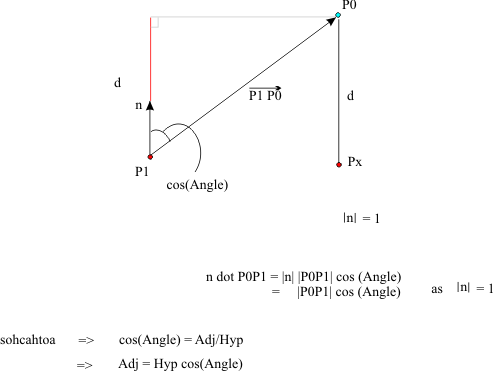Thursday February 2, 2023
 home | about | contact | DonationsThe Maths of 3D You can't have 3D without a little maths...
 p {margin-top:0; margin-bottom:0;} Minimum Distance From A Point To A Plane Tutorial by bkenwright@xbdev.netBoth methods generate the same solution, but its how you think about solving it....one method uses a parametric line view of it, the other things more in terms of planes and the plane equation.   ...Using Method 1....   o Step 1.   Lets make some assumptions first, n is our plane normal, and its normalised.  So |n|=1.        P1 to P0 = P1P0 = P0-P1   And P1 is a point on our plane. d is the shortest distance from our point P0 to the plane   o Step 2.This gives us:     d = n dot |P0 - P1|   o Step 3.   Now d is a distance, its a value, not a vector.  So we still need to determine the point Px on the plane.  We use our n direction value to know which way where going, and combine it with an amplitude so we know how far to go.  (Not forgetting P0 as our starting point)       Px = P0 + d*n   where:     d = n dot |P0-P1|     ....Method 2.... For this second method, we sort of work with a non-normalised plane vector and the plane equation.As before, our point P0, and we want to find the closest distance to the plane.  Our plane is defined by P1, a ponit on the plane (x,y,z) and the planes normal n. Now for a plane, any point on the plane must satisfy the plane equation, which is:   A x + B y + C z + D = 0   The minimum distance is then the absolute value of:      ( A x0 + B y0 + C z0 + D ) / sqrt( A2 + B2 + C2 )     But ackk...what is that D value?   D is the distance from the plane to the origin.       minimum distance = d = (A (x0 - x1) + B (y0 - y1) + C (z0 - z1)) / sqrt(A2 + B2 + C2)                                       = ( A x0 + B y0 + C z0 + D ) / sqrt(A2+B2+C2)                                       = [  (n dot P0) - (n dot P1) ] / sqrt(A2+B2+C2)                                       = n dot (P0 - P1) /  sqrt(A2+B2+C2)     We don't have a normalised normal n for the second method example, which is why we divide by |n|.

 Visitor: 9534626 { 229.27.38.75 } Copyright (c) 2002-2020 xbdev.net - All rights reserved. Designated articles, tutorials and software are the property of their respective owners.